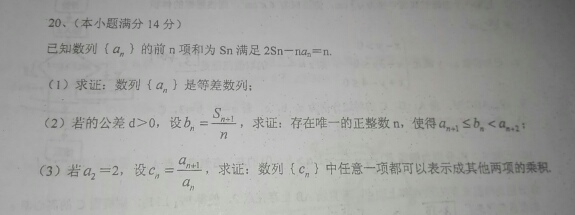| APP | 校园号 | 客服 客服热线：400-863-9889（2）由题意可得：当n=1时，2S1-a1=1，解得a1=1．可得Sn+1bn=
 Sn+1 n
，代入an+1≤bn＜an+2即可证明．
（3）由（1）可得：数列{an}是等差数列；又a1=1，a2=2，可得an=n，cn=
 an+1 an
=
 n+1 n
，对于∀n∈N*，设cn=ck•cl，其中k，l∈∈N*．k≠n，l≠n．
 n+1 n
=
 k+1 k
 l+1 l
，化简整理即可证明．

n≥2时，（n-1）an-1-（n-2）an=1，

∴数列{an}是等差数列．
（2）由题意可得：当n=1时，2S1-a1=1，解得a1=1．

 (n+1)n 2
d，∴bn=
 Sn+1 n
=
(n+1)+
 n(n+1) 2
d
n

∴1+nd≤
(n+1)+
 n(n+1) 2
d
n
＜1+（n+1）d，

n2-n-
 2 d
≤0
n2+n-
 2 d
＞0
，解得
1+
 8 d
-1
2
＜n≤
1+
 8 d
+1
2

1+
 8 d
+1
2
＞0．
1+
 8 d
+1
2
-
1+
 8 d
-1
2
=1．

（3）由（1）可得：数列{an}是等差数列；又a1=1，a2=2，
∴公差d=1，
∴an=1+n-1=n，
cn=
 an+1 an
=
 n+1 n

 n+1 n
=
 k+1 k
 l+1 l

 1 n
=（1+
 1 k
）（1+
 1 l
）．
∴k=
 n(l+1) l-n

∴数列{cn}中任意一项cn，都存在l=n+1，k=n（n+2）．使得cn=ck•cl